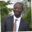Related Tags

filter
sort
javascript
communitycreator

# How to use filter() to find the union of two arrays in JavaScriptAbel Lifaefi Mbula

### Overview

If there are two sets, A and B, then the new set, C, made of elements in both A and B, is called a union in set theory in mathematics. It is denoted as A U B.

### Venn diagram

The Venn diagram for the union looks like the following:

The Venn diagram for the union

We will learn to use the filter() method to create a function that takes two arrays representing two sets of elements and returns an array of all unique elements in the two submitted arrays. This is what we call the union, as introduced at the start of this shot.

### Example

Our input is made of two arrays of integers:

• arr1 = [2, 4, 6, 8, 10]
• arr2 = [1, 2, 3, 4, 5]

The expected output is:

• The union arr3 = arr1 U arr2 = [1, 2, 3, 4, 5, 6, 8, 10].

### Code

We know something about the union in mathematics. Now is the time to practice by implementing it in JavaScript. Considering the above examples, let's create two arrays of color strings that we will be using as a test example:

const arr1 = [2, 4, 6, 8, 10]
const arr2 = [1, 2, 3, 4, 5]

Now, let's create the base function for our program:

function unionArr(arr1, arr2) {
const arr3 = []

return arr3
}
The base function

We'll mainly use the filter() method. In addition to it, we'll also use concat() and includes():

• filter(): This creates a new array with all elements that pass a given test.
• concat(): This merges arrays.
• includes(): This checks if an array contains a given value/element.

The syntax for filter() looks like this: filter(callbackFn) where callbackFn is a function to test each element of the array. Let's implement this function.

First, let's define what we want to implement. Our goal here is to have a new array of arr2 elements that don't exist in arr1. Concretely, if we consider our example, the function should return [1, 3, 5]. Let's move to the code:

// input arrays
const arr1 = [2, 4, 6, 8, 10]
const arr2 = [1, 2, 3, 4, 5]

function isNotInArr1(elt) {
return !arr1.includes(elt)
}

// output array
console.log(arr2.filter(isNotInArr1))
The filter() method callback function

As we can see, the function is just running as expected.

• Line 6: We use includes().
• Line 10: We use filter() with the callback function.

Now, we concatenate this new array with arr1.

//previous code here
const arrFiltered = arr2.filter(isNotInArr1)

const unionArr = arr1.concat(arrFiltered)

• Line 2: The new array created by filter() is stored in arrFiltered .
• Line 4: The final array created with concat() is stored in unionArr .

### Calculating the union

Let's put everything together and see how things look:.

// input arrays
const arr1 = [2, 4, 6, 8, 10]
const arr2 = [1, 2, 3, 4, 5]

// callback function
function isNotInArr1(elt) {
return !arr1.includes(elt)
}

function unionArr(arr1, arr2) {
const arrFiltered = arr2.filter(isNotInArr1)
const unionArr = arr1.concat(arrFiltered)

// sort the result
return unionArr.sort((a,b) => a - b)
}
// output array
console.log(unionArr(arr1, arr2))
unionArr()

Line 15: We return the array already sorted using sort().

RELATED TAGS

filter
sort
javascript
communitycreator

CONTRIBUTORAbel Lifaefi Mbula
RELATED COURSES

View all Courses

Keep Exploring

Learn in-demand tech skills in half the time